Ex 11.2

Chapter 11 Class 12 Three Dimensional Geometry (Term 2)
Serial order wise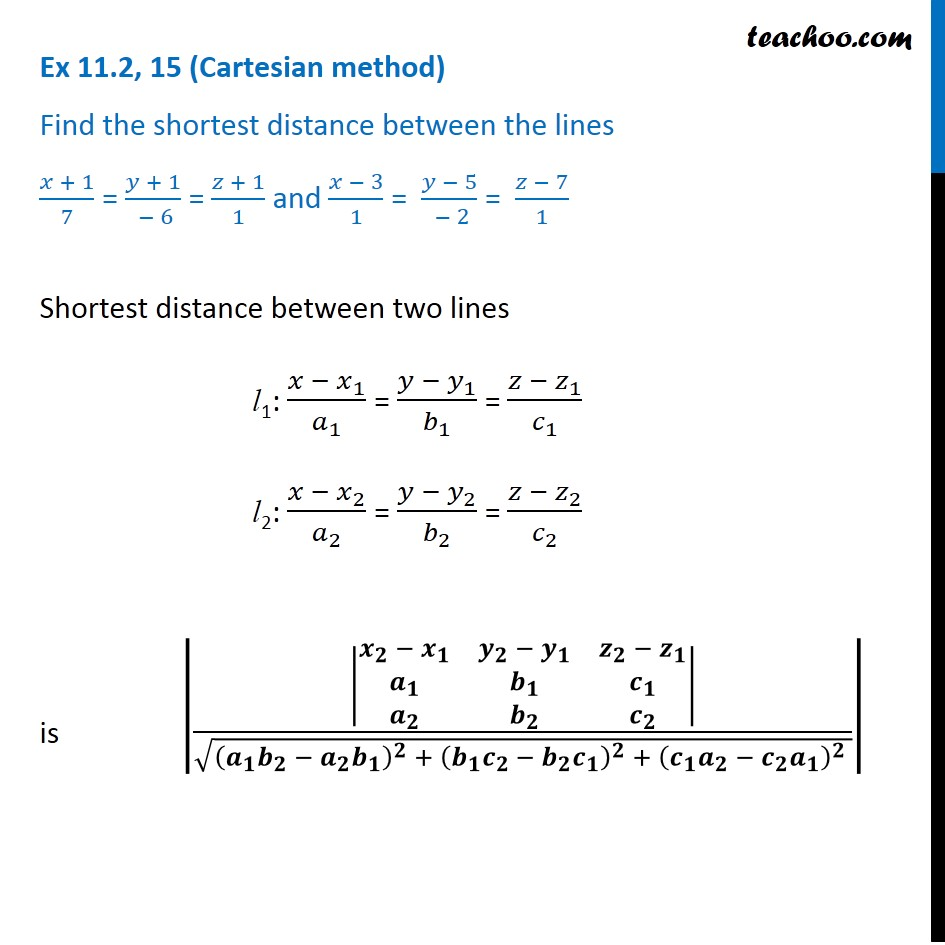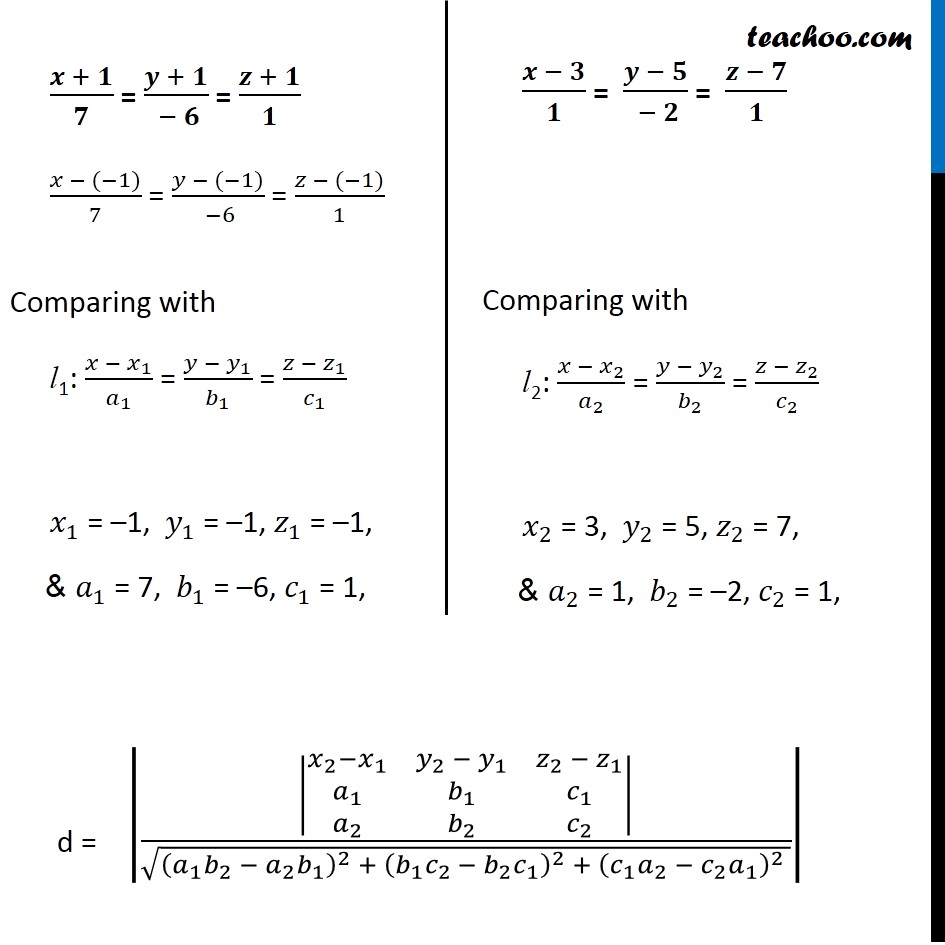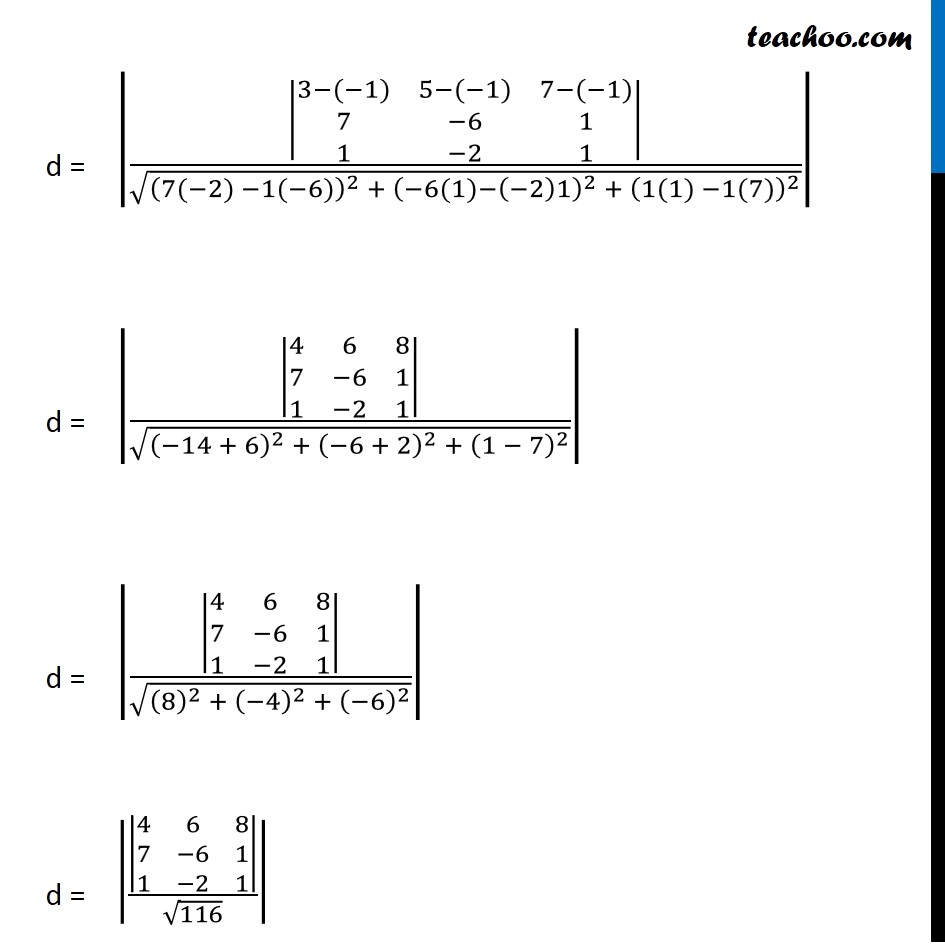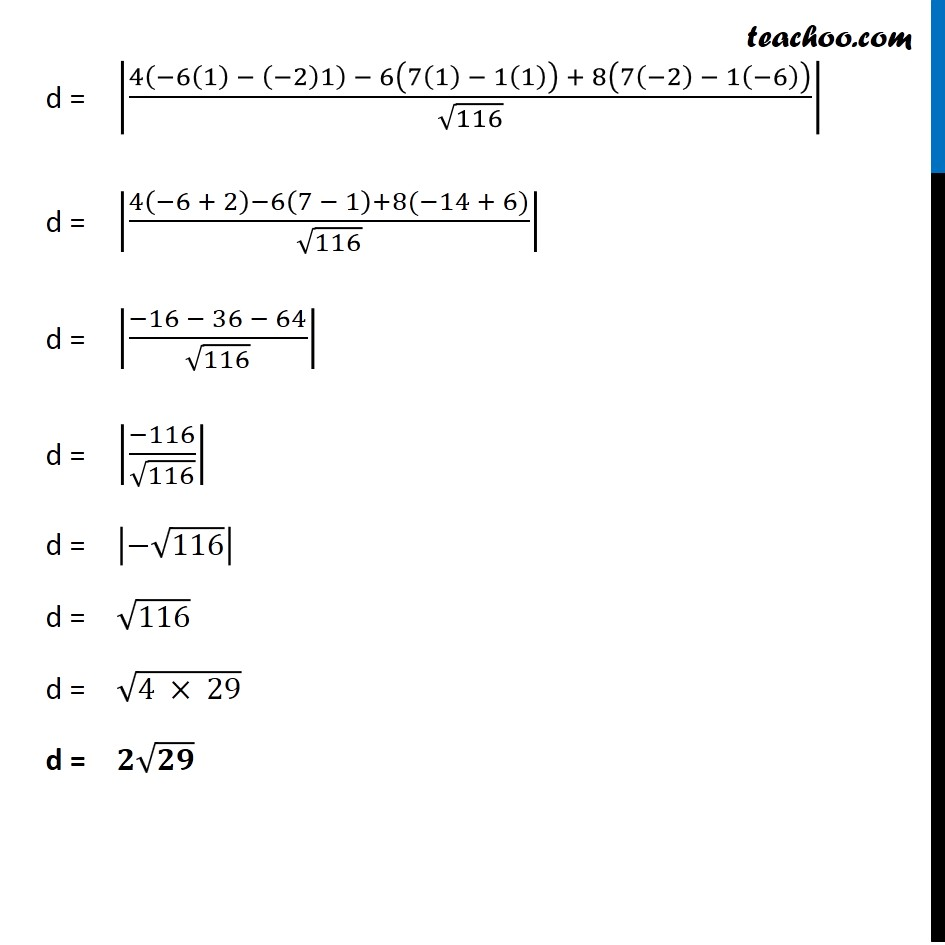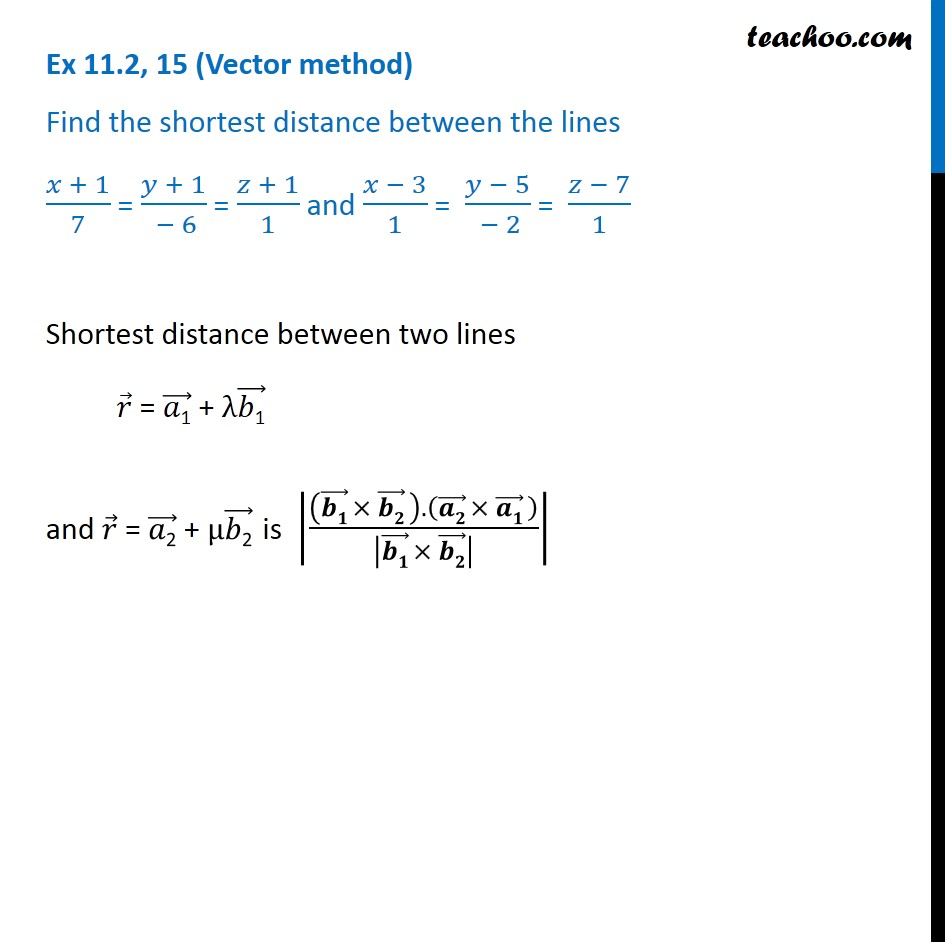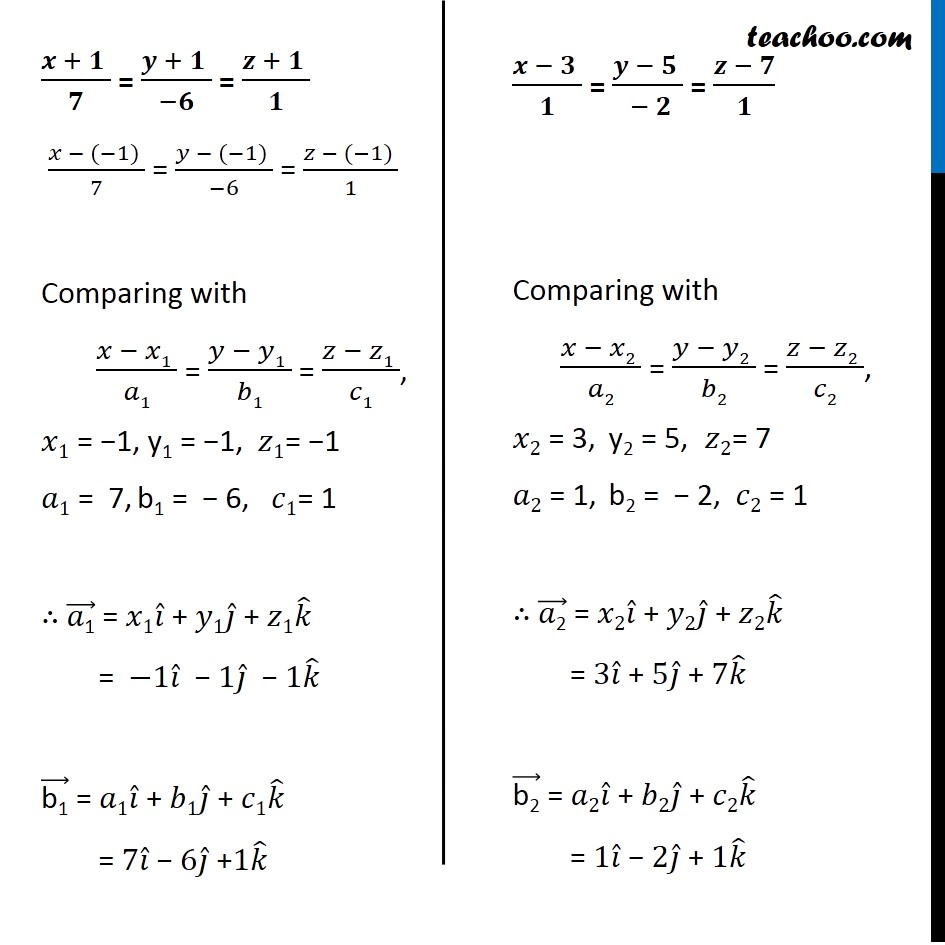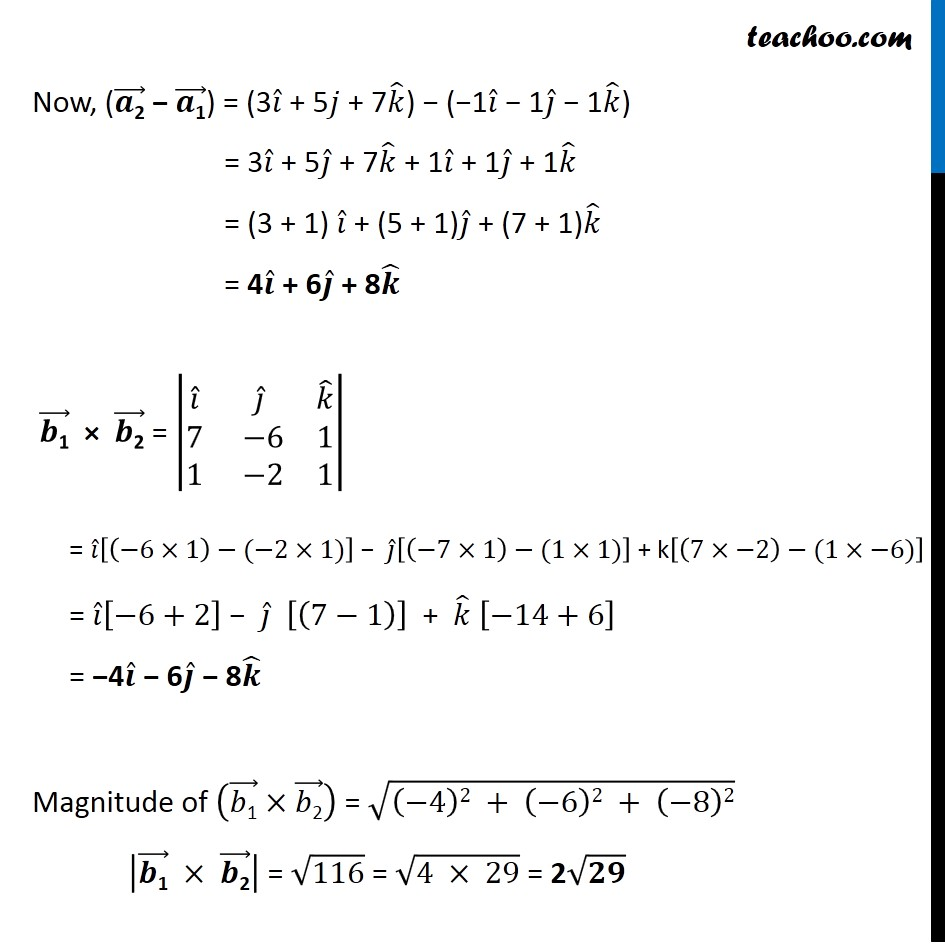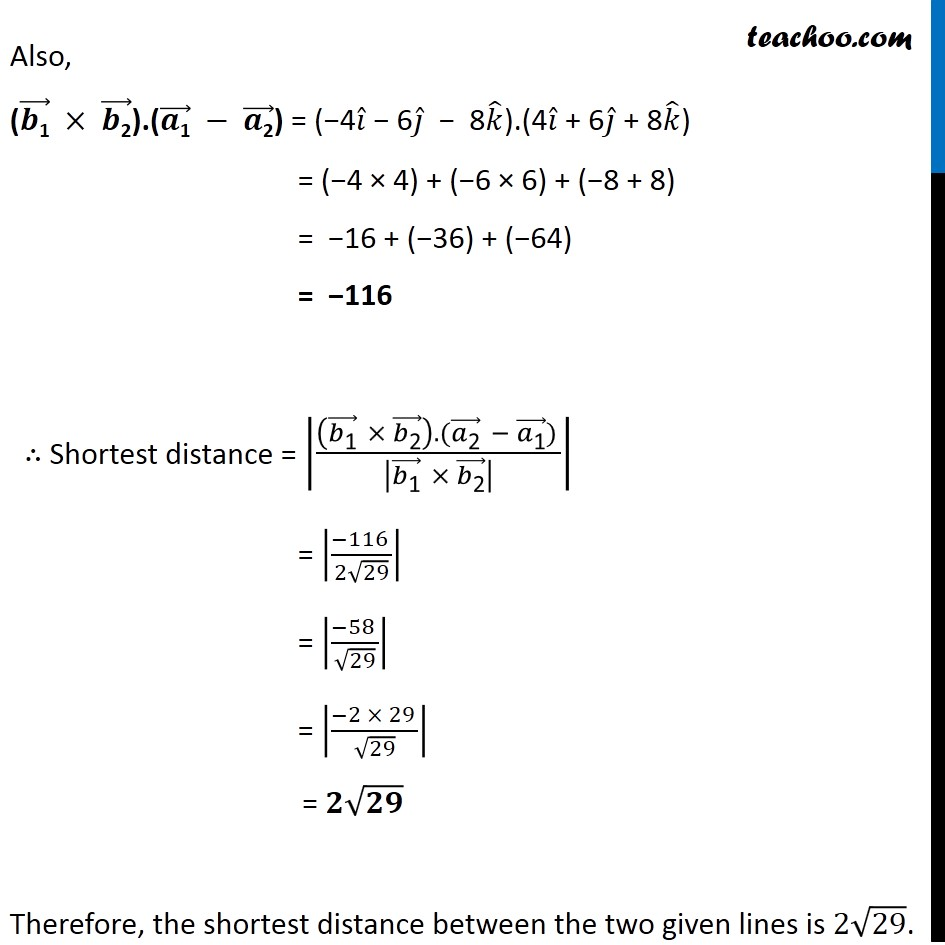Now learn Economics at Teachoo for Class 12

### Transcript

Ex 11.2, 15 (Cartesian method) Find the shortest distance between the lines (𝑥 + 1)/7 = (𝑦 + 1)/( − 6) = (𝑧 + 1)/1 and (𝑥 − 3)/1 = (𝑦 − 5)/( − 2) = (𝑧 − 7)/1 Shortest distance between two lines l1: (𝑥 − 𝑥_1)/𝑎_1 = (𝑦 − 𝑦_1)/𝑏_1 = (𝑧 − 𝑧_1)/𝑐_1 l2: (𝑥 − 𝑥_2)/𝑎_2 = (𝑦 − 𝑦_2)/𝑏_2 = (𝑧 − 𝑧_2)/𝑐_2 is ||■8(𝒙_𝟐 − 𝒙_𝟏&𝒚_𝟐 − 𝒚_𝟏&𝒛_𝟐 − 𝒛_𝟏@𝒂_𝟏&𝒃_𝟏&𝒄_𝟏@𝒂_𝟐&𝒃_𝟐&𝒄_𝟐 )|/√((𝒂_𝟏 𝒃_𝟐 − 𝒂_𝟐 𝒃_𝟏 )^𝟐 + (𝒃_𝟏 𝒄_(𝟐 )− 𝒃_𝟐 𝒄_𝟏 )^𝟐 + (𝒄_𝟏 𝒂_𝟐 −〖 𝒄〗_𝟐 𝒂_𝟏 )^𝟐 )| (𝒙 + 𝟏)/𝟕 = (𝒚 + 𝟏)/( − 𝟔) = (𝒛 + 𝟏)/𝟏 (𝑥 − (−1))/7 = (𝑦 − (−1))/( −6) = (𝑧 − (−1))/1 Comparing with l1: (𝑥 − 𝑥_1)/𝑎_1 = (𝑦 − 𝑦_1)/𝑏_1 = (𝑧 − 𝑧_1)/𝑐_1 𝑥_1 = –1, 𝑦_1 = –1, 𝑧_1 = –1, & 𝑎_1 = 7, 𝑏_1 = –6, 𝑐_1 = 1, (𝒙 − 𝟑)/𝟏 = (𝒚 − 𝟓)/( − 𝟐) = (𝒛 − 𝟕)/𝟏 Comparing with l2: (𝑥 − 𝑥_2)/𝑎_2 = (𝑦 − 𝑦_2)/𝑏_2 = (𝑧 − 𝑧_2)/𝑐_2 𝑥_2 = 3, 𝑦_2 = 5, 𝑧_2 = 7, & 𝑎_2 = 1, 𝑏_2 = –2, 𝑐_2 = 1, d = ||■8(𝑥_2−𝑥_1&𝑦_2 − 𝑦_1&𝑧_2 − 𝑧_1@𝑎_1&𝑏_1&𝑐_1@𝑎_2&𝑏_2&𝑐_2 )|/√((𝑎_1 𝑏_2 − 𝑎_2 𝑏_1 )^2 + (𝑏_1 𝑐_(2 )− 𝑏_2 𝑐_1 )^2 + (𝑐_1 𝑎_2 −〖 𝑐〗_2 𝑎_1 )^2 )| d = ||■8(3−(−1)&5−(−1)&7−(−1)@7&−6&1@1&−2&1)|/√((7(−2) −1(−6))^2 + (−6(1)−(−2)1)^2 + (1(1) −1(7))^2 )| d = ||■8(4&6&8@7&−6&1@1&−2&1)|/√((−14 + 6)^2 + (−6 + 2)^2 + (1 − 7)^2 )| d = ||■8(4&6&8@7&−6&1@1&−2&1)|/√((8)^2 + (−4)^2 + (−6)^2 )| d = ||■8(4&6&8@7&−6&1@1&−2&1)|/√116| d = |(4(−6(1) − (−2)1) − 6(7(1) − 1(1)) + 8(7(−2) − 1(−6)))/√116| d = |(4(−6 + 2)−6(7 − 1)+8(−14 + 6))/√116| d = |(−16 − 36 − 64)/√116| d = |(−116)/√116| d = |−√116| d = √116 d = √(4 × 29) d = 𝟐√𝟐𝟗 Ex 11.2, 15 (Vector method) Find the shortest distance between the lines (𝑥 + 1)/7 = (𝑦 + 1)/( − 6) = (𝑧 + 1)/1 and (𝑥 − 3)/1 = (𝑦 − 5)/( − 2) = (𝑧 − 7)/1 Shortest distance between two lines 𝑟 ⃗ = (𝑎"1" ) ⃗ + λ(𝑏"1" ) ⃗ and 𝑟 ⃗ = (𝑎"2" ) ⃗ + μ(𝑏"2" ) ⃗ is |(((𝒃𝟏) ⃗ × (𝒃𝟐) ⃗ ).((𝒂𝟐) ⃗ × (𝒂𝟏) ⃗ ))/|(𝒃𝟏) ⃗ × (𝒃𝟐) ⃗ | | Ex 11.2, 15 (Vector method) Find the shortest distance between the lines (𝑥 + 1)/7 = (𝑦 + 1)/( − 6) = (𝑧 + 1)/1 and (𝑥 − 3)/1 = (𝑦 − 5)/( − 2) = (𝑧 − 7)/1 Shortest distance between two lines 𝑟 ⃗ = (𝑎"1" ) ⃗ + λ(𝑏"1" ) ⃗ and 𝑟 ⃗ = (𝑎"2" ) ⃗ + μ(𝑏"2" ) ⃗ is |(((𝒃𝟏) ⃗ × (𝒃𝟐) ⃗ ).((𝒂𝟐) ⃗ × (𝒂𝟏) ⃗ ))/|(𝒃𝟏) ⃗ × (𝒃𝟐) ⃗ | | (𝒙 + 𝟏 )/𝟕 = (𝒚 + 𝟏 )/(−𝟔) = (𝒛 + 𝟏 )/𝟏 (𝑥 − (−1) )/7 = (𝑦 − (−1) )/(−6) = (𝑧 − (−1) )/1 Comparing with (𝑥 − 𝑥1 )/𝑎1 = (𝑦 − 𝑦1 )/𝑏1 = (𝑧 − 𝑧1 )/𝑐1, 𝑥1 = −1, y1 = −1, 𝑧1= −1 𝑎1 = 7, b1 = − 6, 𝑐1= 1 ∴ (𝑎"1" ) ⃗ = 𝑥1𝑖 ̂ + 𝑦1𝑗 ̂ + 𝑧1𝑘 ̂ = −1𝑖 ̂ − 1𝑗 ̂ − 1𝑘 ̂ ("b1" ) ⃗ = 𝑎1𝑖 ̂ + 𝑏1𝑗 ̂ + 𝑐1𝑘 ̂ = 7𝑖 ̂ − 6𝑗 ̂ +1𝑘 ̂ (𝒙 − 𝟑 )/𝟏 = (𝒚 − 𝟓 )/( − 𝟐) = (𝒛 − 𝟕)/𝟏 Comparing with (𝑥 − 𝑥2 )/𝑎2 = (𝑦 − 𝑦2 )/𝑏2 = (𝑧 − 𝑧2 )/𝑐2, 𝑥2 = 3, y2 = 5, 𝑧2= 7 𝑎2 = 1, b2 = − 2, 𝑐2 = 1 ∴ (𝑎"2" ) ⃗ = 𝑥2𝑖 ̂ + 𝑦2𝑗 ̂ + 𝑧2𝑘 ̂ = 3𝑖 ̂ + 5𝑗 ̂ + 7𝑘 ̂ ("b2" ) ⃗ = 𝑎2𝑖 ̂ + 𝑏2𝑗 ̂ + 𝑐2𝑘 ̂ = 1𝑖 ̂ − 2𝑗 ̂ + 1𝑘 ̂ Now, ((𝒂"2" ) ⃗ − (𝒂"1" ) ⃗) = (3𝑖 ̂ + 5𝑗 + 7𝑘 ̂) − (−1𝑖 ̂ − 1𝑗 ̂ − 1𝑘 ̂) = 3𝑖 ̂ + 5𝑗 ̂ + 7𝑘 ̂ + 1𝑖 ̂ + 1𝑗 ̂ + 1𝑘 ̂ = (3 + 1) 𝑖 ̂ + (5 + 1)𝑗 ̂ + (7 + 1)𝑘 ̂ = 4𝒊 ̂ + 6𝒋 ̂ + 8𝒌 ̂ (𝒃"1" ) ⃗ × (𝒃"2" ) ⃗ = |■8(𝑖 ̂&𝑗 ̂&𝑘 ̂@7& −6&1@1& −2&1)| = 𝑖 ̂[(−6×1)−(−2×1)] − 𝑗 ̂[(−7×1)−(1×1)] + k[(7×−2)−(1×−6)] = 𝑖 ̂[−6+2] − 𝑗 ̂ [(7−1)] + 𝑘 ̂ [−14+6] = −4𝒊 ̂ − 6𝒋 ̂ − 8𝒌 ̂ Magnitude of ((𝑏"1" ) ⃗×(𝑏"2" ) ⃗ ) = √((−4)2 + (−6)2 + (−8)2) |(𝒃"1" ) ⃗" " ×" " (𝒃"2" ) ⃗ | = √116 = √(4 × 29) = 2√𝟐𝟗 Also, ((𝒃"1" ) ⃗ ×" " (𝒃"2" ) ⃗).((𝒂"1" ) ⃗" "−" " (𝒂"2" ) ⃗) = (−4𝑖 ̂ − 6𝑗 ̂ − 8𝑘 ̂).(4𝑖 ̂ + 6𝑗 ̂ + 8𝑘 ̂) = (−4 × 4) + (−6 × 6) + (−8 + 8) = −16 + (−36) + (−64) = −116 ∴ Shortest distance = |(((𝑏"1" ) ⃗ × (𝑏"2" ) ⃗ ).((𝑎"2" ) ⃗ − (𝑎"1" ) ⃗) )/|(𝑏"1" ) ⃗ × (𝑏"2" ) ⃗ | | = |(−116 )/(2√29)| = |(−58 )/√29| = |(−2 × 29 )/√29| = 𝟐√𝟐𝟗 Therefore, the shortest distance between the two given lines is 2√29.